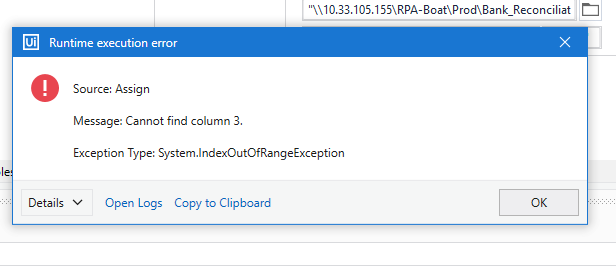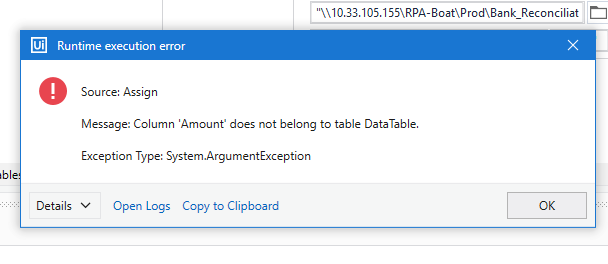# Excel key

HI Team,
i want to do sum of my D column so how i can do

Use Assign activity and create the Variable as System.Double

Use this expression in the RHS in Assign activity

``````(From d in dtData.AsEnumerable Where Not (isNothing(d(3)) OrElse String.IsNullorEmpty(d(3).toString.Trim)) Select v = CDbl(d(3).toString.Trim)).Sum(Function (x) x)
``````

Regards
Gokul

Use Assign activity and create the Variable as System.Double
var=dt.AsEnumerable.Sum(function(x)cdbl(x(“which column you want”)))

cheers

@Kuldeep_Pandey

Another method

`dt.AsEnumerable.Sum(function(x) if(IsNumeric(x("Amount").ToString),CDBL(x("Amount").ToString),0)).ToString`

Or

``````dt.Compute("Sum(Amount)", string.Empty).ToString
``````

cheers

Not working

Its not finding column amount

Do you have any error? @Kuldeep_PandeyCheck out the XAML file

SumExcelColumn.xaml (16.1 KB)

Try with this Instead of 3 you can use Amount in the expression and check it

Regards
Gokul

@Kuldeep_Pandey

Column index starts from 0 …so if its column 3 then index will be 2

cheersError
Can we have simple expression to add Column D

Have you use Read range activity? With Header enable in the properties?

Regards
Gokul

Sorry There is range issue
thanks
kuldeep

This topic was automatically closed 3 days after the last reply. New replies are no longer allowed.